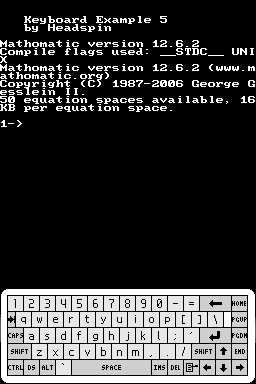# MathomaticDS

### From GameBrew

MathomaticDSGeneral
AuthorDavr
Last Updated2006/11/11
TypeMath
Version2006
Website
Source

Mathomatic is a portable, general purpose CAS (Computer Algebra System) that can symbolically solve, simplify, combine, and compare algebraic equations, perform complex number and polynomial arithmetic, etc. It does some calculus and handles all algebra, except trigonometry and logarithms.

Mathomatic is entirely hand-written in optimized C. All arithmetic is double precision floating point with 14 decimal digits accuracy.

## Features

• Combine and solve algebraic equations.
• Completely simplify and compare expressions.
• Perform calculus transformations.
• Help with series analysis.
• Perform standard, complex number, and polynomial arithmetic.
• Generate efficient C, Java, or Python code from equations.

## User guide

User manual is provided in the file Doc/.

## Credits

Thanks to WinterMute for ​devkitpro.

Thanks to HeadKaze for his keyboard.